# Machine Learning in Medicine

##### The Clinical Research Unit provides a blue print for using machine learning in medicine
Method & Analytics Team - by Zara Aminolroaya, under supervision of Cord Lethebe

The applications of machine learning (ML) to address medical field problems and the healthcare industry are increasing day by day. The Clinical Research Unit has provided the following article, which explains the difference between machine learning and statistics, steps to use machine learning, and different applications of machine learning in the medical field.

##### Intro - Machine Learning vs. Statistics

Machine learning is making healthcare smarter, but old-school statistics still has its place in healthcare analytics. There are different criteria for choosing between machine learning or statistical analytics, such as the analytics goal, the size of data, the number of variables, etcetera.

Machine learning and statistical learning are nearly related in many aspects and are more or less the same, but they consider different perspectives on the same problem:

Statistics: Statistics is defined as the study of collection, analysis, interpretation, presentation, and organization of data.
Machine Learning: Machine learning gives computers the ability to learn without being explicitly programmed.

The following is a comparison of these two techniques based on the purpose of data analysis.

Machine LearningStatistical Modeling
• Emphasize prediction
• Emphasize results via prediction performance
• Concern for overfitting but not model complexity
• Emphasis on performance
• Generalizability is obtained through performance on novel datasets
• Usually no superpopulation model specified
• Concern over performance and robustness
• Used for problems with many variables
• Used when Dataset is big
• Emphasize superpopulation inference/explanation
• Focus on a-priori hypothesis
• Simpler models preferred over complex ones (parsimony), even if the more complex models perform slightly better
• Emphasis on parameter interpretability
• Statistical modeling or sampling assumptions connects data to a population of interest
• Concern over performance and robustness
• Used for problems with few variables
• Used when Dataset is small

Statistical models are often easier to interpret, and they are suitable for the description of the biological relationships when the features have mainly an additive effect. Machine learning is suitable for prediction and decision making about new data. ML is promising in the areas that are not traditional “tabular data”, such as images.

##### Formulation

The formulation of machine learning and statistical modeling is different even with the same end goal.

In a statistical model, we basically try to estimate the function f in:
Dependent Variable ( Y ) = f(Independent Variable) + error function

Machine Learning takes away the deterministic function “f” out of the equation. It simply becomes:
Output(Y) ----- > Input (X)

It will try to find pockets of X in n dimensions (where n is the number of attributes), where occurrence of Y is significantly different.

##### Language

A major difference between statistics and machine learning is their languages. Some terms can have similar meaning in statistics and machine learning but with different languages:

Machine LearningStatistics
Sample/ instanceSubject/ observation
TargetOutcome Variable
LearningEstimation
Example/ instanceData point
Supervised LearningRegression
SoftmaxMultinomial Regression
Supervised LearningClassification
FeatureCovariates
LabelResponse
Graph/ networkModel
WeightsParameters
GeneralizationTest set performance
LossLog-liklihood
NoiseMeasurement error
One-hot encodingDummy coding
PrecisionPositive predictive value
Confusion MatrixContingency table
GroundtruthGold standard
##### Big vs. Small Data

For better performance, machine learning models need more data than statistical models. Powerful predictive models, such as neural networks and random forests, usually use datasets in the scales of thousands and millions for suitable performance. In contrast, statistical models often can infer and make predictions on hundreds of observations

##### Many vs. Few Variables

Machine learning models select between variables based on their relevance to the outcome, but statistical models are generally not like ML models. In fact, when there are more predictor variables than observations (for example, when using many genes’ status as predictors), statistical models fail completely, while machine learning models proceed unphased.

##### Machine Learning Step by Step

When using machine learning for addressing a problem, it is important to become familiar with different areas in ML. Each area includes different topics: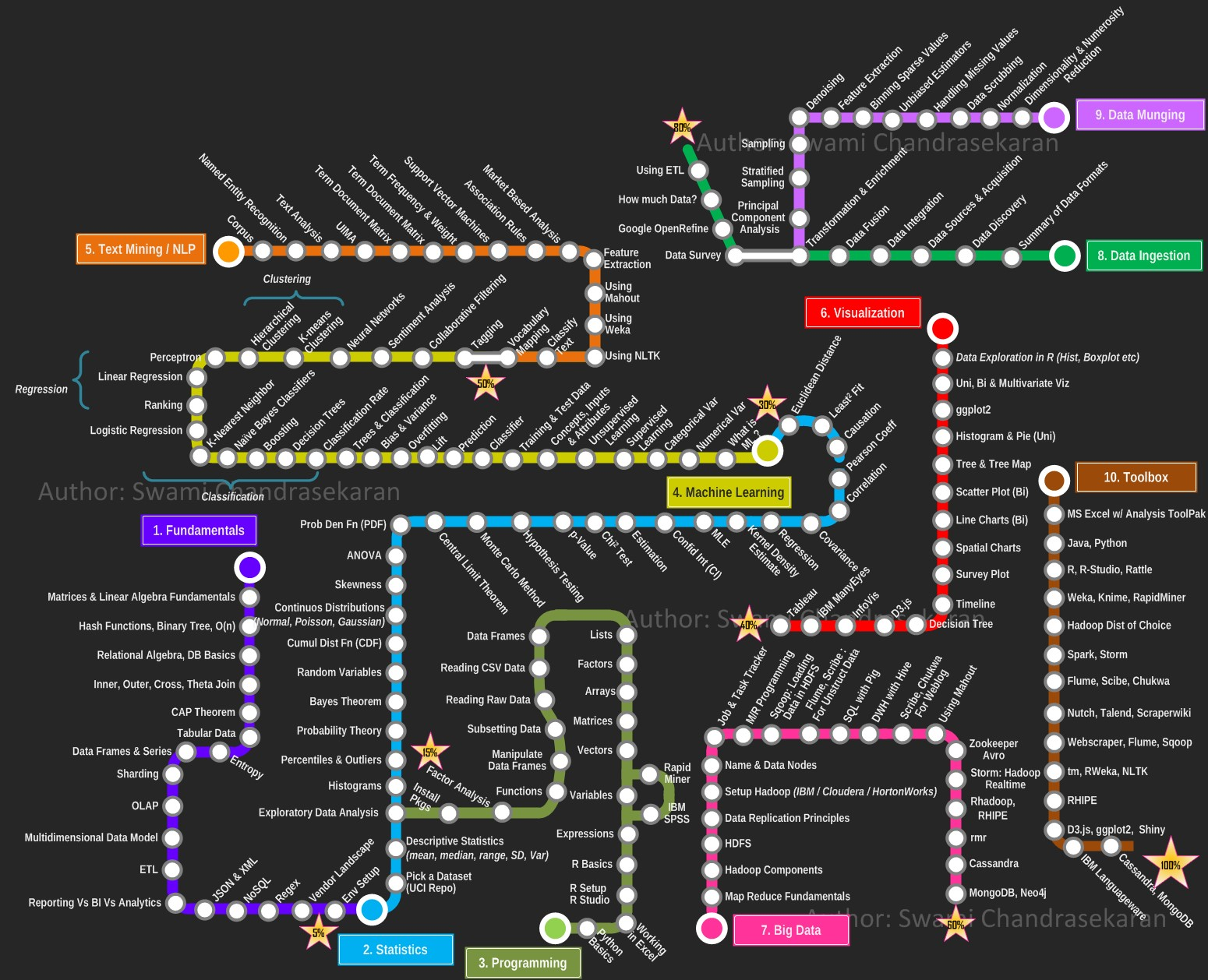Now, let's look at one of the road maps to apply machine learning for addressing a specific problem. Choosing a suitable machine learning algorithm for the problem depends on different factors, such as the nature of the data, computational time, etc.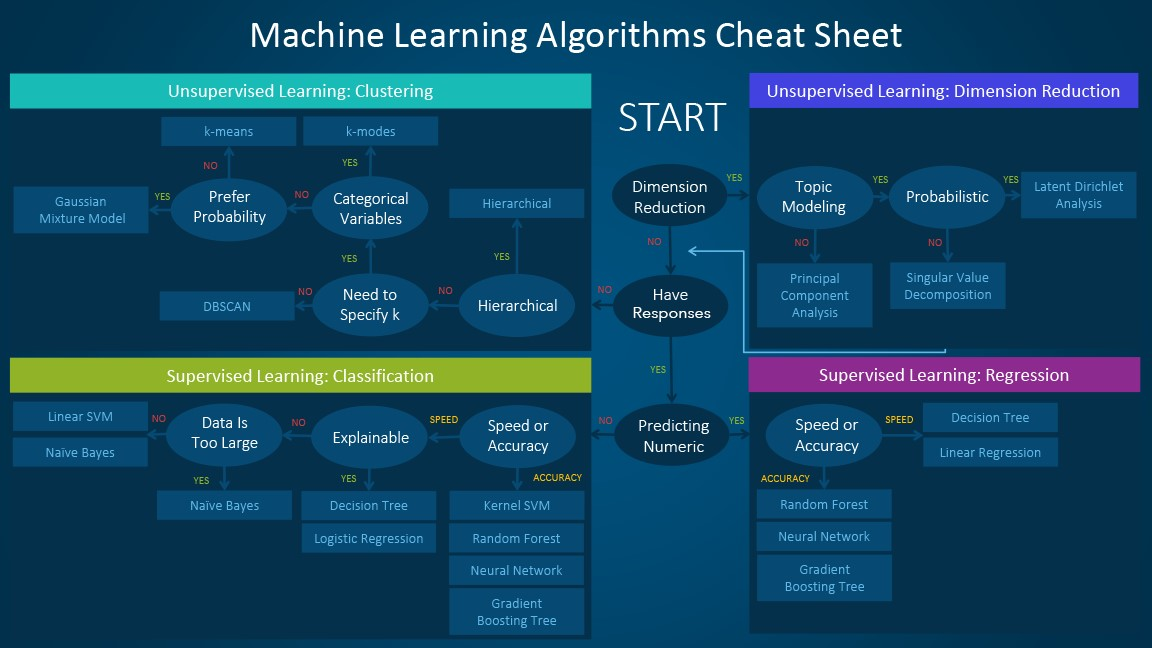The following sections discuss important concepts relating to different steps for choosing machine learning algorithms.

#### Supervised Learning vs. Unsupervised Learning

##### Supervised Learning

In supervised learning, a labeled dataset is ready for training. In fact, it is known what the outcome should look like.

Supervised Learning problems are broadly categorized as regression and classification problems.

###### Regression Problem

In a regression problem, results are predicted within a continuous output, meaning that we are trying to map input variables to some continuous function. The metrics that are commonly minimized/maximized in the model fit stage for regression problems are the Mean Squared Error (MSE), Mean Absolute Error (MAE), R2, etc.

Most popular regression algorithms are:

• Linear Regression
• Logistic Regression
• Polynomial Regression
• Stepwise Regression
• Ridge Regression
• Lasso Regression
• ElasticNet Regression

###### Classification Problem

In a classification problem, input variables are into discrete categories. A classification model attempts to draw some conclusions from observed values. Given one or more inputs, a classification model will try to predict the value of one or more outcomes. The metrics that are commonly minimized/maximized in classification problems are the misclassification rate, Area Under the Receiver Operating Characteristic curve (AUROC or AUC), F1-score, sensitivity, specificity, etc.

There are two types of classification analysis:

1. Binomial Classification
2. Multiclass Classification

Binomial Classification

Binary or binomial classification is the task of classifying the elements of a given set into two groups
Popular algorithms: Lasso Logistic Regression, Decision trees, Random Forest, Bayesian networks, Support vector machines, Neural networks

Multiclass Classification

Multiclass classification is a classification task with more than two classes.
Popular algorithms: Multinomial logit, Decision trees, Random Forest, Bayesian networks, Support vector machines, Neural networks, Nearest neighbor

The following image shows the difference between classification and regression: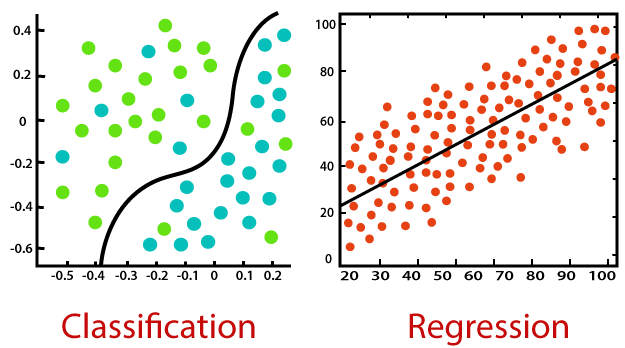##### Unsupervised Learning

Unsupervised learning is a type of machine learning algorithm used to draw inferences from datasets consisting of input data without labeled responses. The most common unsupervised learning method is cluster analysis, which is used for exploratory data analysis to find hidden patterns or grouping in data. Also, dimension reduction is considered as unsupervised learning, in which the variables of data are reduced based on the relationships between datasets.

###### Clustering Algorithms

Most popular clustering algorithms are:

• Partitioning methods
• Hierarchical clustering
• Fuzzy clustering
• Density-based clustering
• Model-based clustering

The below image shows the difference between hierarchical and non-hierarchical clusterings.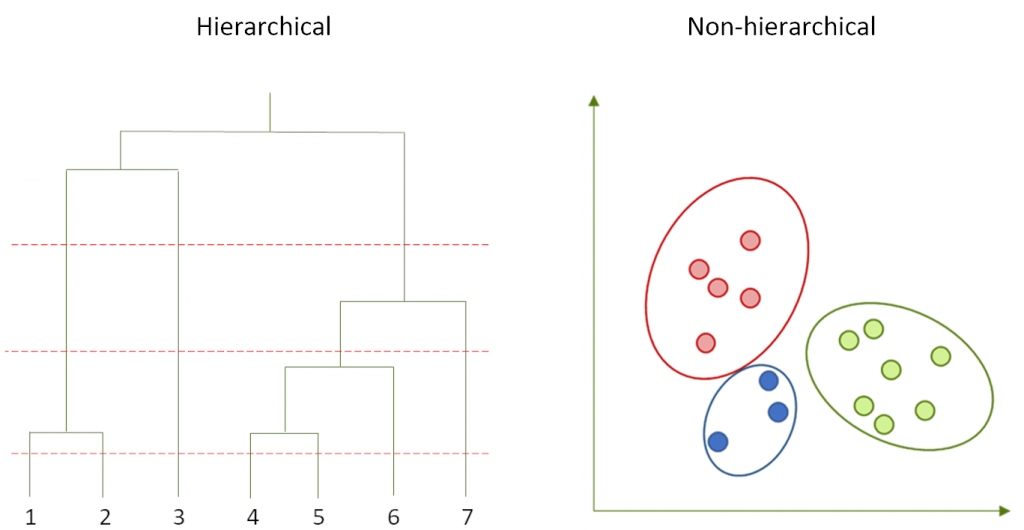###### Dimensional Reduction

Some variables (features) are redundant or irrelevant according to the prediction. With the dimension reduction, the true relationship between features and the outcome can be identified.

##### Description

Polynomial regression is a form of regression analysis in which the relationship between the independent variable x and the dependent variable y is modelled as an nth degree polynomial in x.

##### Data Requirement

Obervations must be independant

##### Complexity

Calling 𝑛 the number of observations and p the number of weights, the overall complexity should be 𝑛^2 𝑝+ 𝑝^3. Execution time of the algorithms is highly related to different factors such as Hardware, optimization, etc. for example, in Rule Based Systems in a Distributed Environment, they could run the logistic regression algorithms for 100 GB data with 100 machine for less than 70 s.

##### Interpretability

Regressions are interpretable. The relationship between x and y is totally observable.

##### Description

Lasso (least absolute shrinkage and selection operator; also Lasso or LASSO) is a regression analysis method that performs both variable selection and regularization in order to enhance the prediction accuracy and interpretability of the statistical model it produces.

##### Data Requirement

Lasso regression puts constraints on the size of the coefficients associated to each variable. However, this value will depend on the magnitude of each variable. It is therefore necessary to center and reduce, or standardize, the variables.

##### Complexity

Let the number of candidate variables (features, columns) be 𝐾 and the sample size (number of observations, rows) be 𝑛. Consider LASSO implemented using LARS algorithm. The computational complexity of LASSO is 𝑘^3+ 𝑘^2 𝑛.

##### Interpretability

Interpretability decreases if the target is dependent on lot of features

##### Description

K-means algorithms can be used to subdivide data points of a dataset into clusters based on nearest mean values. To determine the optimal division of your data points into clusters, such that the distance between points in each cluster is minimized, k-means clustering would be appropriate algorithms. In the term k-means, k denotes the number of clusters in the data.

##### Data Requirement

The type of data best suited for K-Means clustering would be numerical data with a relatively lower number of dimensions.

##### Complexity

K-mean is NP hard problem. O(kn) for each iteration where k = no. of cluster, n = no. of points. For example based on An Improved Mini Batch K-means Algorithm Based on Mapreduce with Big Data the running time for 1 million data is 100 S or for 9 million is about 400 S.

##### Interpretability

Groups of data are recognizable after clustering.

##### Description

Logistic regression is the appropriate regression analysis to conduct when the dependent variable is dichotomous (binary).  Like all regression analyses, the logistic regression is a predictive analysis. Logistic regression is used to describe data and to explain the relationship between one dependent binary variable and one or more nominal, ordinal, interval or ratio-level independent variables.

##### Data Requirement

Logistic regression does not require a linear relationship between the dependent and independent variables. Also, the error terms (residuals) do not need to be normally distributed. Finally, the dependent variable in logistic regression is not measured on an interval or ratio scale.

##### Complexity

Complexity of training for logistic regression methods with gradient based optimization: O((f+1)csE), where:

• f - number of features (+1 because of bias). Multiplication of each feature times it's weight (foperations, +1 for bias). Another f + 1 operations for summing all of them (obtaining prediction). Using gradient method to improve weights counts for the same number of operations, so in total we get 4* (f+1) (two for forward pass, two for backward), which is simply O(f+1).
• c - number of classes (possible outputs) in your logistic regression. For binary classification it's one, so this term cancels out. Each class has it's corresponding set of weights.
• s - number of samples in your dataset, this one is quite intuitive I think.
• E - number of epochs you are willing to run the gradient descent (whole passes through dataset)

Note: this complexity can change based on things like regularization (another c operations), but the idea standing behind it goes like this.

##### Interpretability

The formulation of logistic regression in terms of log odds is the fundamental reason why logistic regression coefficients aren't interpretable.  logistic regression isn't completely a black box: The linearity assumption means that you can compare the relative impact of the covariates by their coefficients, (assuming you've appropriately scaled the covariates). And the impact of changing a covariate depends only upon current estimated probability and the magnitude of the change, (in more complex models it can depend on the current values of all of the covariates).

##### Description

A decision tree is a decision support tool that uses a tree-like model of decisions and their possible consequences, including chance event outcomes, resource costs, and utility.

##### Data Requirement

Decision trees can handle both categorical and numerical data.

##### Complexity

Let 𝑁 = number of training examples, 𝑘= number of features, and 𝑑 = depth of the decision tree. The time complexity for decision trees is in 𝑂(𝑁𝑘𝑑) .

##### Interpretability

It is highly interpretable. Different Decisions are observable.

##### Description

A neural network is a series of algorithms that endeavors to recognize underlying relationships in a set of data through a process that mimics the way the human brain operates.

##### Data Requirement

Need a large large dataset.

##### Complexity

The complexity for learning 𝑚 examples, where each gets repeated 𝑒 times, and where 𝑤 is the number of weights, is (𝑤∗𝑚∗𝑒).

##### Interpretability

Neural networks are not considered to be interpretable.

##### Description

A Support Vector Machine (SVM) is a discriminative classifier formally defined by a separating hyperplane. In other words, given labeled training data (supervised learning), the algorithm outputs an optimal hyperplane which categorizes new examples.

##### Data Requirement

Data should be numerical.

##### Complexity

Linear SVM has prediction complexity 𝑂(𝑑) with 𝑑 is the number of input dimensions since it is just a single inner product. The followings illustrate SVM algorithms runt time based on the number of datasets.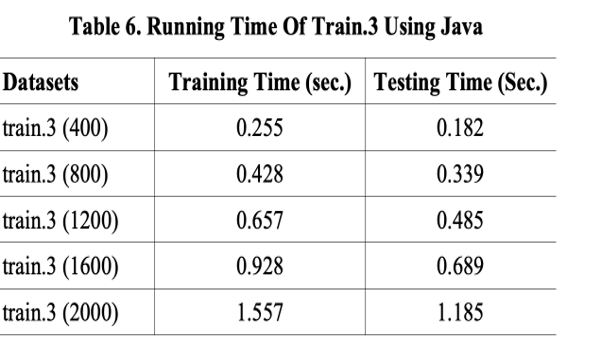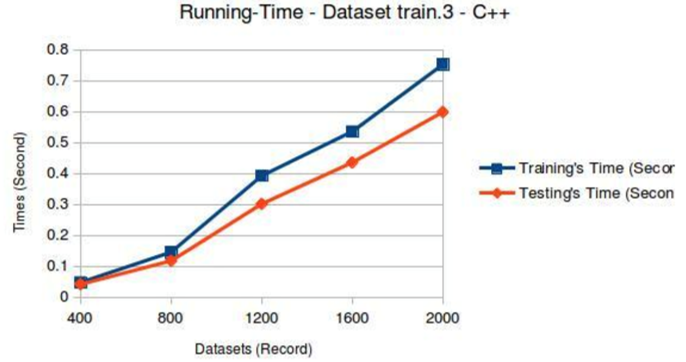##### Interpretability

Linear SVMs are also interpretable as any other linear model, since each input feature has a weight that directly influences the model output. Non-linear SVMs are partially interpretable, as they tell you which training data are relevant for prediction, and which aren't. This is not possible for other methods such as Random Forests or Deep Networks.

##### Description

Bayesian networks are a type of probabilistic graphical model that uses Bayesian inference for probability computations. Bayesian networks aim to model conditional dependence, and therefore causation, by representing conditional dependence by edges in a directed graph. Through these relationships, one can efficiently conduct inference on the random variables in the graph through the use of factors.

##### Data Requirement

Data should be categorical.

It is NP hard.

##### Interpretability

Bayesian networks are visually interpretable.

##### Tools
Some machine learning and data mining tools that require minimal experience include:
For working with more powerful tools as below, some data mining and programming experience is required:
##### Interpretability Vs. Accuracy

There is a tradeoff between the predictive accuracy of a model and how easy the model is to interpret. For example, linear regression is a simple model with a few parameters which is easy to interpret. However, it may not have sufficient predictive power for particular use cases. On the other hand, models like neural networks with millions of parameters will often highly perform for prediction. However, these complex models do not always make business sense and it would be hard to explain to clients how models made decisions.

It is important to deliver a project to a business client and build confidence in the algorithmic approaches. There are different ways to increase the understandability of machine learning results for a client user. One of them is to explain how different inputs affect the model's performance.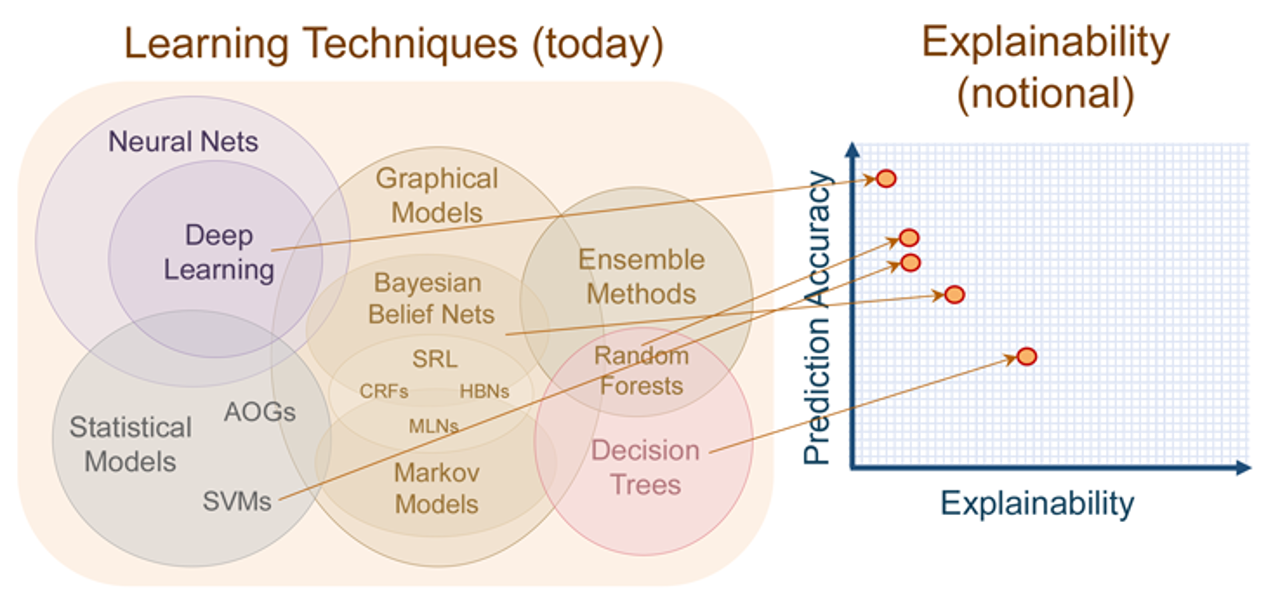#### Research Purpose

There are different research purposes in the medicine field, and machine learning proposes different techniques to solve problems in each area:

##### Diagnostic

Medical diagnosis is the process of determining which disease or condition explains a person's symptoms and signs. Machine learning is being used to diagnose cancer, pneumonia, and other diseases, and with enough reliable datasets, it is often more accurate and faster at diagnosis than real doctors. Diagnostic problems can be solved by classification methods. For example, the below image shows the high algorithm performance on detecting the lung cancer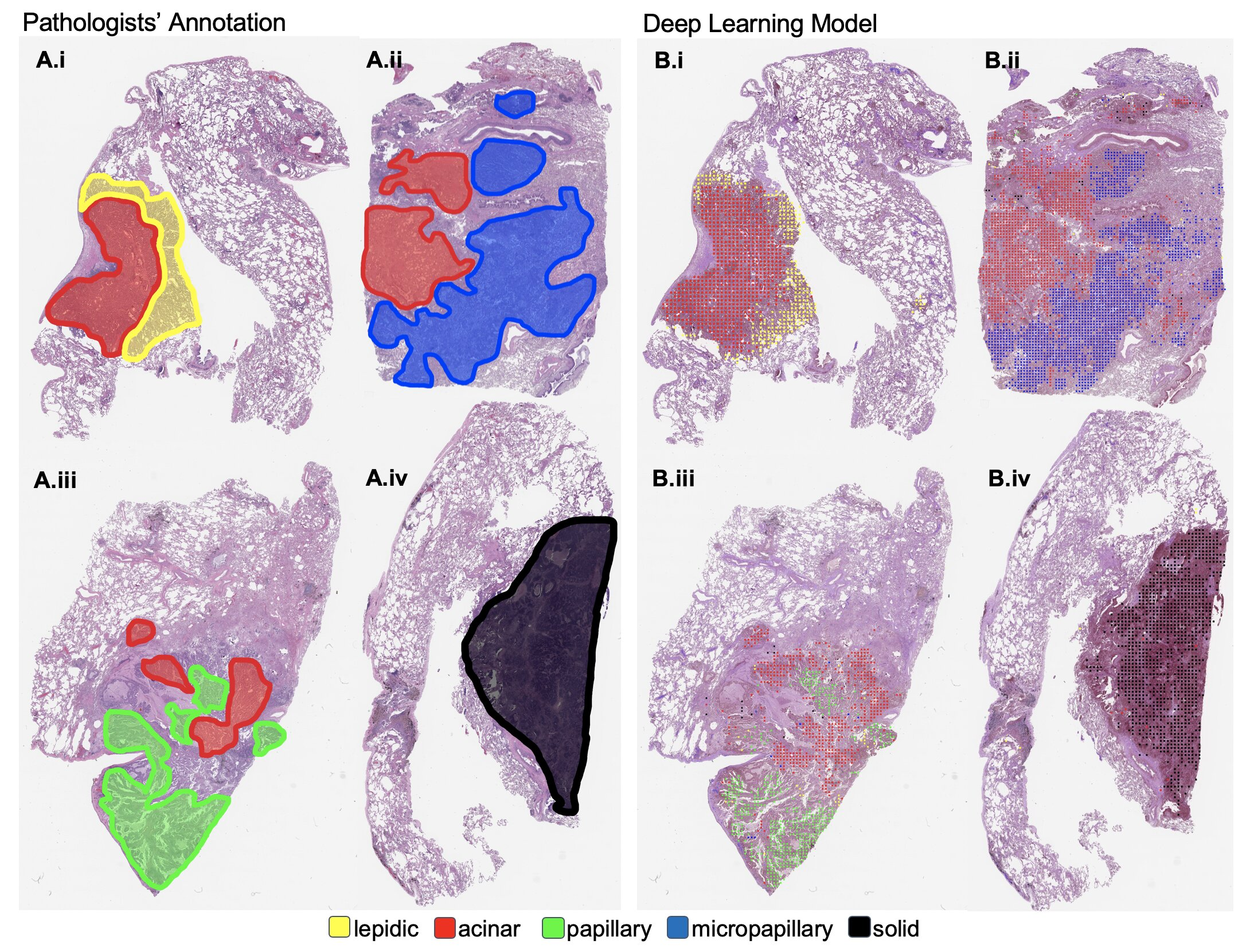##### Prediction

Predictive analytics is the process of learning from historical data in order to make predictions about the future (or any unknown). For health care, predictive analytics will enable the best decisions to be made. Prediction problems can be solved by classification or regression methods.

##### Frequency

Frequency is used for epidemiological measurements to describe the occurrence of disease. Frequency problems can be solved by regression methods.

##### Association

Association is a statistical relationship between two or more events, characteristics or other variables - e.g., an association between exposure to X and a health effect, Y - which may not imply cause and effect. Association problems can be solved by regression methods.

##### Finding Similar Groups

Clustering can be used for finding similar groups of different elements and it is the process of analyzing, examining relationships in, and organizing theoretically the current knowledge in a field of study in order to add to an existing knowledge base and generate further questions for research. Clustering methods can be used for finding similar groups. For example, the below image shows the heatmap analysis of microarray data showing hierarchical clustering of differentially expressed genes.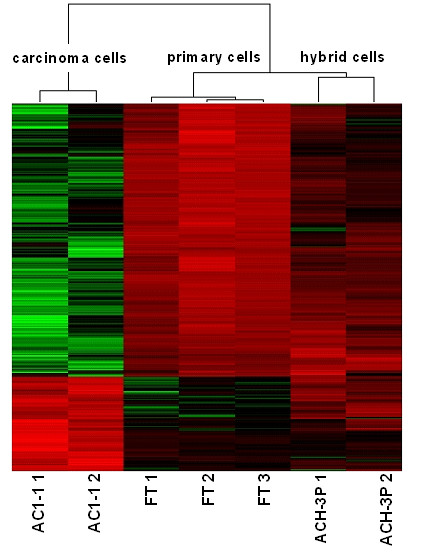#### Health Data Types

The number of digitized health and wellness data increases day by day, which makes the opportunity for analyzing data through data science and machine learning methods. For data analysis, due to the complexity of the human condition, the data related to a patient would often be retrieved and integrated from multiple sources and should be analyzed from different aspects. Combining data from various resources, such as hospitals, clinics, etc., contributes to earning valuable knowledge. With the increase of the data size, some big data approaches should be considered in conjunction with machine learning algorithms to solve a problem.

In the following, there is an organization of data types relating to different health and wellness sources.##### Summary

In the medicine field, it is common to use statistical analytics and machine learning to solve problems based on a problem target and data features. This article looks at different concepts of machine learning and refers to different areas in ML. Finally, the article explains the use of machine learning techniques based on the research purposes in the medicine field.

Email Us